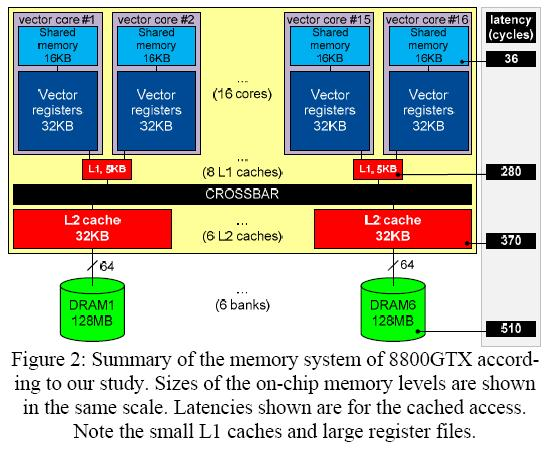# latency of shared memory of Tesla C1060

Dear all:

From Volkov’s paper,

Vasily Volkov, James W. Demmel, Benchmarking GPUs to Tune Dense Linear Algebra. In SC â€™08: Preceedings of the 2008 ACM/IEEE conference on Supercomputing.

Piscataway, NJ, USA, 2008, IEEE Press.

( can be obtained in the thread http://forums.nvidia.com/index.php?showtopic=89084 )

authors show that latency of shared memory of 8800GTX is 36 cycle, see figure 1

figure 1:I try to find latency of shared memory of Tesla C1060, and the experimental result shows

latency of shared memory is 34 cycle.

The procedures of my experiment is:

I modify code in cuda_latency.tar.gz provided by @Sylvain Collange in the thread

http://forums.nvidia.com/index.php?showtop…rt=#entry468968

The kernel is

``````#define   SMEM_SIZE  256

static __global__ void smem_latency(int * data,  timing_stats * timings)

{

__shared__ float b[SMEM_SIZE];

volatile unsigned int start_time = 1664;

volatile unsigned int end_time = 1664;

#pragma unroll 1

for (int i = 0; i < SMEM_SIZE; ++i){

b[i] = data[i];

}

int k = 0;

for( int j = 0; j < 2; j++){

start_time = clock();

#pragma unroll

for (int i = 0; i < SMEM_SIZE; ++i){

k = b[k];

}

end_time = clock();

}

if ( 0 == threadNum ){

timings.start_time = k;

}

}
``````

and execution configuration is 1 grid and 1 thread

``````dim3  grid(1, 1, 1);

``````

The result is 58 cycle. ( this is bigger than 36 cycle )

However if we use decuda to deassembly .cubin file, then

``````int k = 0;

for( int j = 0; j < 2; j++){

start_time = clock();

#pragma unroll

for (int i = 0; i < SMEM_SIZE; ++i){

k = b[k];

}

end_time = clock();

}

``````

would be decoded as

``````label1: mov.b32 \$r2, %clock

shl.u32 \$r2, \$r2, 0x00000001

movsh.b32 \$ofs1, \$r3, 0x00000002

cvt.rzi.s32.f32 \$r3, s[\$ofs1+0x0020]

movsh.b32 \$ofs1, \$r3, 0x00000002

cvt.rzi.s32.f32 \$r3, s[\$ofs1+0x0020]

movsh.b32 \$ofs1, \$r3, 0x00000002

cvt.rzi.s32.f32 \$r3, s[\$ofs1+0x0020]

...

movsh.b32 \$ofs1, \$r3, 0x00000002

cvt.rzi.s32.f32 \$r3, s[\$ofs1+0x0020]

mov.b32 \$r4, %clock

shl.u32 \$r4, \$r4, 0x00000001

set.ne.s32 \$p0|\$o127, \$r1, c1[0x0004]

@\$p0.ne bra.label label1

bar.sync.u32 0x00000000
``````

we can compare source code with result of decuda and find relationship between them,

``````r1 <-- 0 is variable â€œjâ€

r3 <-- 0 is variabel â€œkâ€

r2 = start_time <--ïƒŸ%clock x 2

\$ofs1 <-- k * sizeof(int)

r3 <-- b[k]

\$ofs1 <-- k * sizeof(int)

r3 <-- b[k]

... ...

r4 = end_time <-- %clock x 2

...
``````

This means that “k = b[k]” has two instructions

``````S1 :  \$ofs1 <-- k * sizeof(int)

S2 :  r3 <-- b[k]
``````

and its Gatt chart is shown in figure 2.

figure 2:

but latency of shared memory should be execution time of instruction 2,

we have known pipeline latency of MAD is 24 cycle, so execution time of S2 is 58 - 24 = 34 cycle,

which is latency of shared memory.